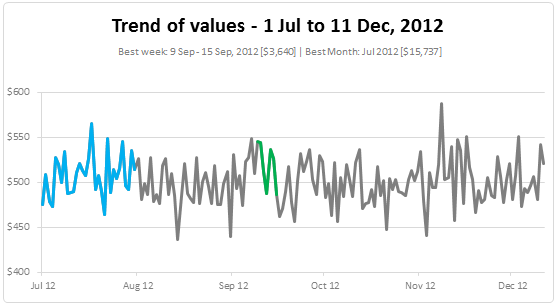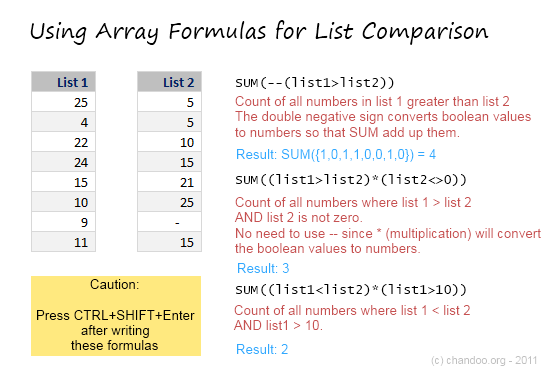# All articles with 'sumif()' Tag

## Introduction to Excel SUMIFS Formula

Published on May 25, 2018 in Learn ExcelExcel SUMIFS function is used to calculate the sum of values that meet any criteria. For example, you can calculate the total sales in east zone for product Pod Gun using SUMIFS formula.

• What is SUMIFS function and how to use it?
• Syntax for SUMIFS
• Using SUMIFS() with tables and structural references
• SUMIFS examples – simple, wild card
• Using SUMIFS() with date & time values
• Free sample file for SUMIFS formula
• More formulas for data analysis
Continue »

## CP007: aweSUM() – Overview of SUM functions in Excel

Published on May 1, 2014 in Chandoo.org Podcast Sessions, Learn ExcelIn the 7th session of Chandoo.org podcast, lets make you aweSUM().

Imagine for a second that Excel cannot add up numbers. And no it cant subtract them either. What would that look like?

A glorified Notepad. That’s right. Excel’s ability to add up numbers, along with features like formulas, charts, pivot tables & BHATTEXT() are what make it such a lovely software. May be not the BHATTEXT(), but we all agree that Excel is so versatile and useful because it can add up numbers (and perform other calculations) with ease.

But how well do you know the SUM formulas of Excel?

In this podcast, you will learn,

• Special personal fruit announcement 😛
• + operator
• Status bar & total rows in tables
• Auto Sum feature
• SUM() function
• SUMIFS function
• Special cases of SUMIFS function
• SUBTOTAL & AGGREGATE functions
• Other summing functions – SUMPRODUCT etc.
Continue »

## Highlight best week & month in a trend chart [tutorials]

Published on Dec 12, 2012 in Charts and GraphsWhen analyzing business data like sales, shop visits or productivity, one of the questions managers always ask is,

What is the best month / week for this data?

To answer this question, we need to make a chart that looks like above. Today, lets learn how to highlight portions of such charts that correspond to best week or best month.

Continue »

## Formula Forensics No. 029 SumIf with Inconsistent Column Layouts

Published on Sep 20, 2012 in Formula Forensics, Huis, Posts by HuiHow do we perform a Sumif on seemingly randomly spaced columns?
Today in Formula Forensics we look at a solution to do just that.

Continue »

## Comparing Lists of Values in Excel using Array Formulas

Published on Jun 14, 2011 in Excel Howtos, Learn ExcelLast week, we had a home work on Calculating Donation Summaries using Excel Formulas. This is a good case where array formulas can help us. So today, we will learn how we can use Array Formulas to compare lists of values and calculate summaries. Towards the end of this post, you can see a video that explains the solution to Donation Summary Calculation problem.

Continue »

## Use Copy & Paste to Preserve References to Tables [Quick Tip]

Published on May 23, 2011 in Learn ExcelWith Excel 2007, Microsoft has introduced a powerful and useful feature called as Tables. One of the advantages of Tables is that you can write legible formulas by using structural references. That means, you can write easy to understand formulas like this,

But, there is a problem. When you write these formula and drag the formula cell sideways to fill remaining cells, Excel changes table column references and thus makes your formulas almost useless.

Well, there is a simple workaround for this problem

Continue »

## 6 Tips for Writing Better VLOOKUPs

Published on Nov 2, 2010 in Learn ExcelOk, you have learned how to write vlookup formulas. You have also seen some pretty interesting examples of it (1, 2).

But how do you write better VLOOKUP formulas?

Here is a list of 6 tips that work wonders with VLOOKUP writing.

Continue »

## How to Look up Based on Multiple Conditions

Published on Nov 2, 2010 in Excel Howtos, Learn ExcelSituationNot always we want to lookup values based on one search parameter. For eg. Imagine you have data like below and you want to find how much sales Joseph made in January 2007 in North region for product “Fast car”? Read more to find how to solve this.

Continue »

## SUMIF works in 2D too [quick tip]

Published on Apr 27, 2010 in Learn ExcelWe all know that SUMIF formula can be used to find the sum of values meeting a criteria. Like this, But I was pleasantly surprised to realize that SUMIF works equally well for 2D ranges too, like this: During a recent consulting work with a client I had a requirement to sum up values that […]

Continue »

## What is Excel SUMPRODUCT formula and how to use it?

Published on Nov 10, 2009 in Featured, Learn ExcelToday we will learn a new and exciting excel formula – the all powerful SUMPRODUCT.

At the outset SUMPRODUCT formula may not seem like all that useful. But once you understand how excel works with lists (or arrays) of data, the SUMPRODUCT’s relevance becomes crystal clear.

Continue »

## Product Recommendation – Excel Lookup Toolbox

Published on Nov 5, 2009 in Learn Excel, productsAnyone working on the data using excel will know the importance of lookup formulas. They are vital for making almost any spreadsheet or dashboard. That is why when my friend John Franco, who maintains Excel-Spreadsheet-Authors.com, wrote to me about his new book Excel lookup toolbox I was truly excited. In this post I am going to share my review of this product.

Continue »

## 29 Excel Formula Tips for all Occasions [and proof that PHD readers truly rock]

Published on Aug 24, 2009 in Excel Howtos, Featured, Learn Excel

It is no exaggeration that knowing excel formulas can give you a career boost. From someone starting at the long list of numbers, you can suddenly become a data god who can lookup, manipulate and analyze any spreadsheet.

So when our little excel blog hit the 5000 RSS Subscriber milestone, I celebrated the occasion by asking you to share an excel formula through twitter or comments with rest of us. And boy, what an excellent list of formula tips you have shared with us all.
Here is the complete list of entries for the twitter formula contest.

Continue »

## Sumif with multiple conditions [quick tip]

Published on Jul 22, 2009 in Excel HowtosHere is a little formula trick if you need to sum a range of cells based on multiple conditions.

Assuming you have the starfleet, captain and flight data, you can use the good old sum() in an array formula to conditionally sum values meeting multiple criteria. Read on to learn this quick tip.

Continue »

## Eight ways to exploit COUNTIF() … and sumif() too [spreadcheats]

Published on Nov 12, 2008 in Learn ExcelIf for every countif() I write excel paid me a dollar, I would be a millionaire by now. It is such a versatile and fun formula to work with that I have decided to write about it as third post in our spreadcheats series. Using COUNTIF() to replace pivot tables: We all know that you […]

Continue »

## Learn Excel IF Formula – 5 Tricks you Didnt know

Published on Jun 9, 2008 in Featured, Learn ExcelExcel has various functions, including functions to calculate inverse cosine of a given value, to multiply 2 matrices, to estimate the internal rate of return. But, most of us(well, just me then..) use just about 5-6 formulas to do our jobs. And IF formulas are a majority of these, so it doesn’t harm to learn […]

Continue »

### Get FREE Excel & Power-BI Newsletter

One email per week with Excel and Power BI goodness. Join 100,000+ others and get it free.